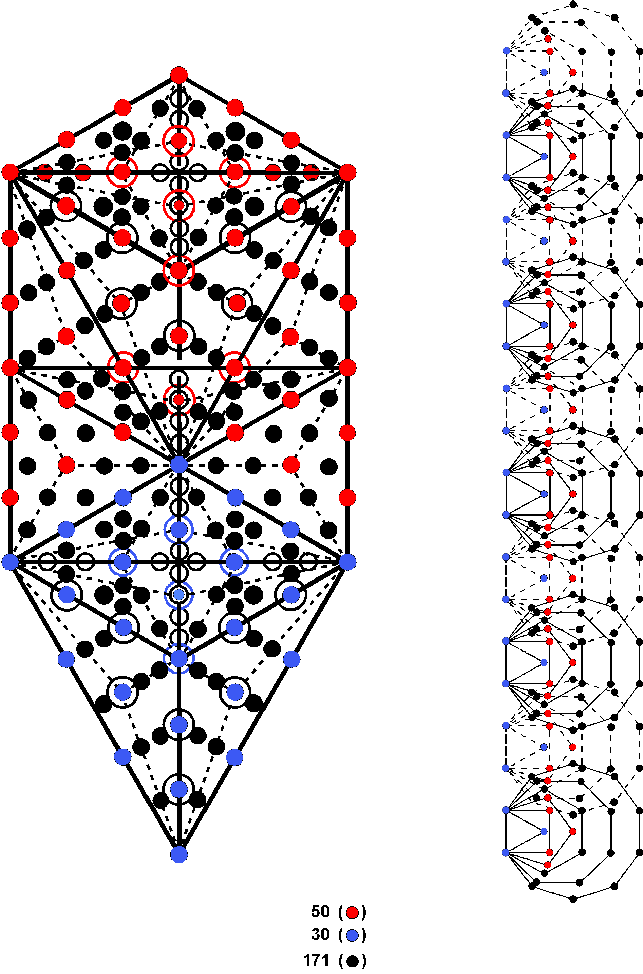<< Previous    1...   6  7    9  10  ...54    Next >>

#8 Correspondence between the 1-tree & the first six polygons enfolded in the 10-treeThe number of corners of the first six types of polygons enfolded in the n-tree is given by:

C(n) = 25n + 1,

where "1" refers to the top corner of the hexagon enfolded in the nth Tree. Therefore, the 60 polygons enfolded in the 10-tree have C(10) = 251 corners. This is the number of yods in the 1-tree when the sectors of its triangles are turned into tetractyses. It demonstrates how the potential replication of a single Tree of Life into 10 such Trees is encoded in its geometry. When its triangles are tetractyses rather than Type A triangles, the 1-tree contains 80 yods made up of the 30 blue yods in its Lower Face and the 50 red yods outside the latter. Conversion of triangles into Type A triangles adds 171 black yods.

The counterpart of these three sets of yods in the 251 corners of the 10 sets of the first six polygons are the 30 blue corners of the triangles, the 50 red corners of the squares & pentagons and the 171 black corners of the hexagons, octagons & decagons.

 << Previous    1...   6  7    9  10  ...54    Next >>

Home